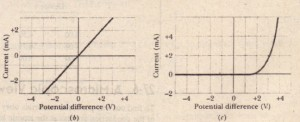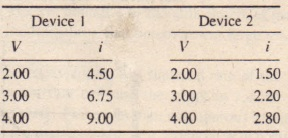# Ohm’s Law

Ohm’s Law

As we just discussed in Section 27·4, a resistor is a conductor with a specified  resistance. It has that same resistance no matter what the magnitude and direction(polarity) of the applied potential difference. Other conducting devices, however, might have resistances that change with the applied potential difference.  shows how to distinguish such devices. A potential difference V is applied across the device being tested, .and the resulting current I through the device is measured as V is varied in both magnitude and polarity. The polarity of V  is arbitrarily taken to be positive when the left terminal of the device is at a higher potential than the right terminal. The direction of the resulting current (from left to right) is arbitrarily assigned a plus sign. The reverse polarity of V (with the right terminal at a higher potential) is then negative; the current it causes is assigned a minus  sign.·llb is a plot of i versus V for one device. This plot is a straight line passing through the origin, so the ratio ilV (which is the slope of the straight line) is the same for all values of V. This means that the resistance R = Vii of the device    independent of the magnitude and polarity of the applied potential difference V.  is a plot for another conducting device. Current can exist in this device only when the polarity of V is p positive and the applied potential difference is more than about 1.5 V. When current does exist, the relation between i and V is not linear; il depends on the value of the applied potential difference VWe distinguish between the two types of device by saying that one obeys Ohm’s law and the other does not.

Ohm’s law is an assertion that the current through a device is always directly proportional to the potential difference applied to the device.

(This assertion is correct only in certain situations; still, for historical reasons, the term “law” is used.) The device of which turns out to be a 1000 n resistor-obeys Ohm’s law. The device of which turns out to be a so-called pin junction diode-does not.

A conducting device obeys Ohm’s law when the resistance of the device is independent of the magnitude and polarity of the applied potential difference

Modern microelectronics-and therefore much of the character of our present technological civilization-depends almost totally on devices that do not obey Ohm’s law. Your calculator, for example, is full of them. It is often contended that V = iR is a statement of  hm’s law. That is not true! This equation is the defining equation for resistance, and it applies to all conducting devices. whether they obey Ohm’s law or not, If we measure the potential difference V across, and the current I through, any device. even a pin junction diode, we can find its resistance at that value of V as R = I’/i. The essence of Ohm’s law, however, is that a plot of i versus V is linear; that is, R is independent of V. We can express Ohm’s law in a more general way if we focus on conducting materials rather than on conducting devices. The relevant relation is then Eq. 27-11 (f = pJ), which is the analog of V = iR.

All homogeneous materials, whether they are conductors like copper or semiconductors like pure silicon or silicon containing special impurities, obey Ohm’s law within some range of values of the electric field. If the field is too strong, however, there are departures from Ohm’s law in all cases

CHECKPOINT

The following table gives the current I (in amperes) through two devices for several values of potential difference I’ (in volts). From these data, determine which device does not obey Ohm’s law